A conversion rate report is one of the more advanced uses of the report designer.

It requires use of many features of the designer including value formulas, Excel style formulas, grouping, and formatting. This tutorial won't go into full detail for each of these topics, but hopefully you'll see how they can be used to get more from your data.

The tutorial assumes that you have a wink reports account, have created an organisation and linked to some connectors, and have a basic knowledge of the report designer. If not, check out some of our other tutorials.

## 1. The plan

For this report, I'll be working with ServiceM8 jobs. Jobs in ServiceM8 can have status of Quote, Work Order, Completed, or Unsuccessful.

I'll be considering a job "converted" if it has status Work Order or Successful, and "not converted" if it has status Unsuccessful. I'm after the conversion rate of last month's jobs (i.e. jobs created last month), and to have that conversion rate broken down by category too.

This style of report isn't just limited to jobs. You might want to track how many invoices are paid on time, how many deliveries are late, or how many employees have filled in their timesheets.

Let's get started!

## 2. Create the report

From the report list for my organisation, I'll use the Design New Report button.I'll call my new report "Job Conversion Rate" and use the Jobs data source.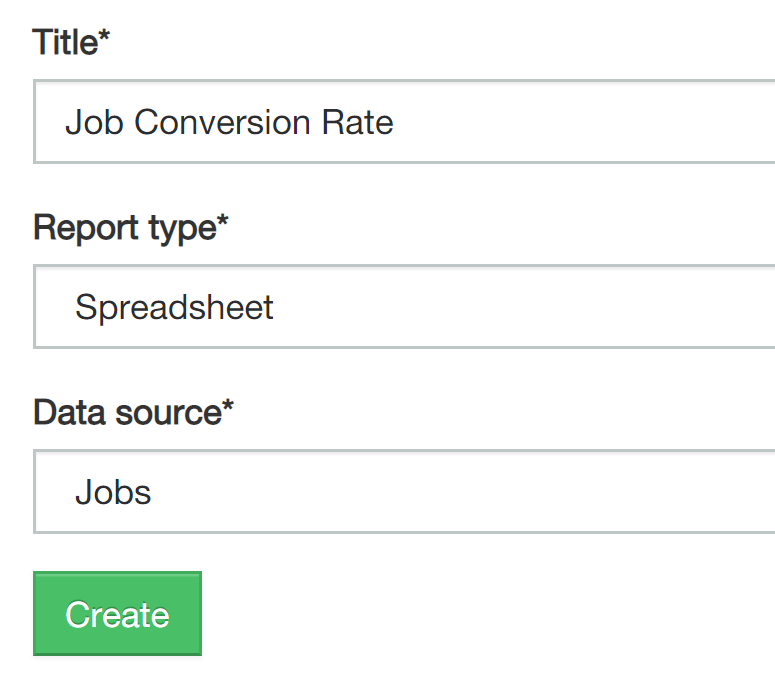I'll assume you've done the tutorial on creating your first report, so will do the next part fairly quickly. I'll choose the Jobs data source and select a date filter.

Remove some of the default column and add some others so that you have this list: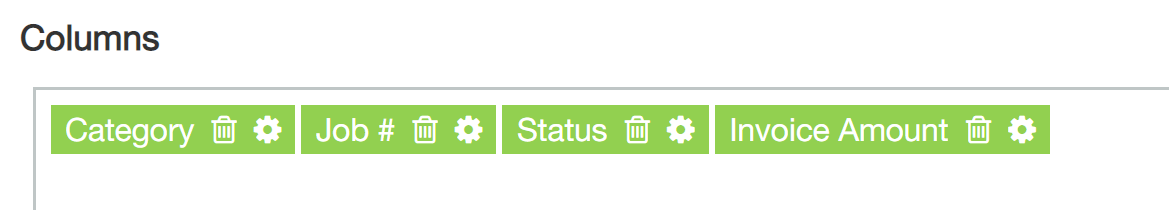Sort by Job #, group by Category:Great! Now we have a list of jobs, grouped into categories, ready for us to apply our conversion rate calculations.

## 3. Add some formula magic

Next we're going to add a few new columns to the report, and use formulas to decide if each job is converted or not.

I'll use the Add Formula Column option to add a new blank column to the report.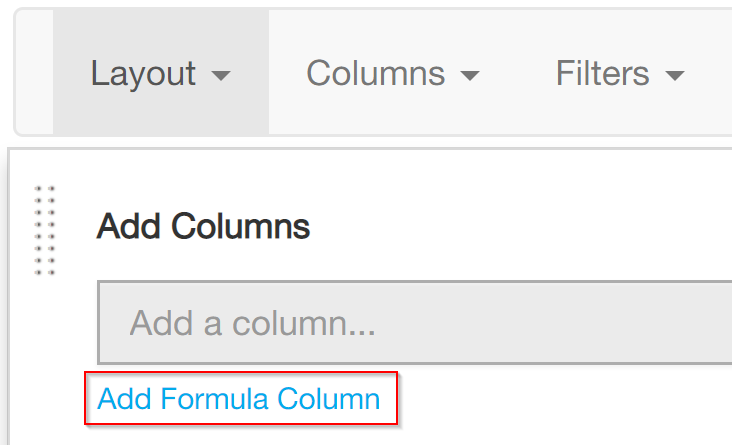I'll rename the new column to be called Is Converted and change the value formula to:

`IF([Status] = "Work Order" OR [Status] = "Completed", 1, 0)`

This formula will make my column value 1 if the status is either Work Order or Completed, and 0 otherwise.

Similarly, I'll add another column called Is Not Converted with value formula:

`IF([Status] = "Unsuccessful", 1, 0)`

Here's what the last columns of my report look like: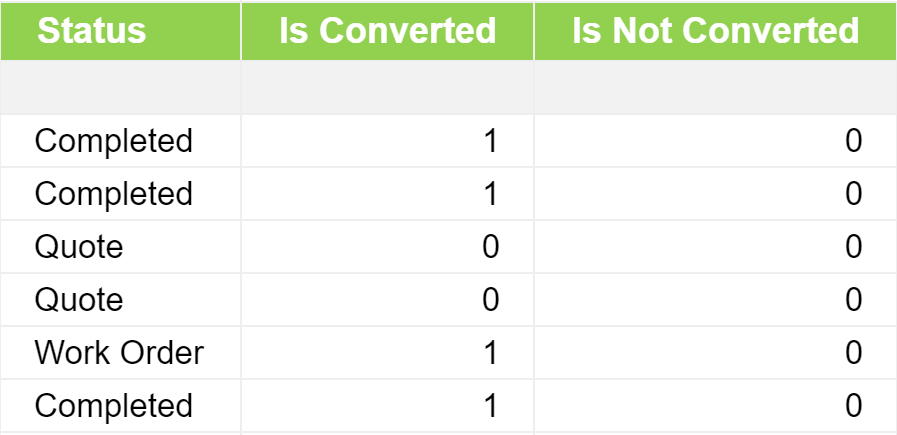And if I set the total row type to Sum for each column, my collapsed report looks like this: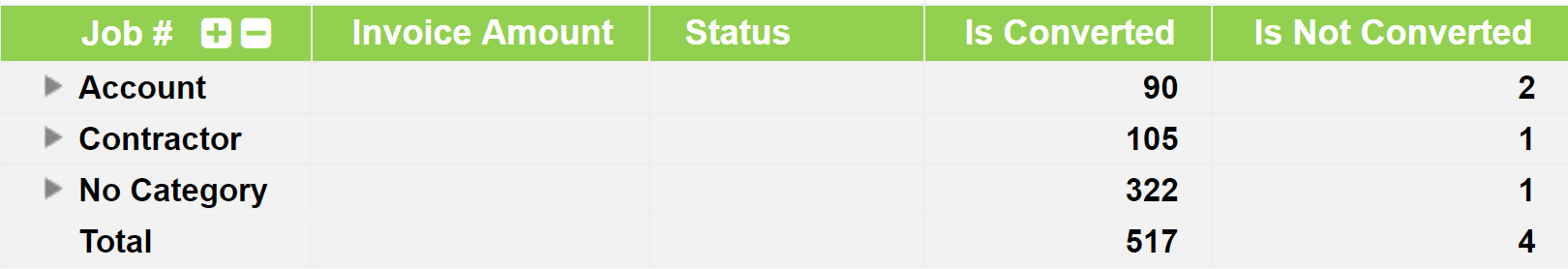This gives us the raw numbers we need to calculate the conversion ratio.

## 4. Percentage formula

The formula for conversion ratio is Total No. Converted / (Total No. Converted + Total No. Not Converted). This formula avoids jobs with Quote status, which don't have a converted status yet.

To use this calculation in a report and especially in the total rows, we need to use an Excel style formula. This special style of formula uses Excel compatible syntax, and is calculated after the rest of the report is populated. It is the only correct way to produce accurate conversion rate totals!

I'm going to add a new column called Conversion Rate, and use the following formula:

`={Is Converted}{row} / ({Is Converted}{row} + {Is Not Converted}{row})`

Note the equals sign at the start of the formula, and the different way of referring to column values. This syntax is covered in the Excel style formula reference.

There's still a few steps until I see the conversion ratio displayed correctly:

• Change the Total Row type for the Conversion Rate column to Formula
• Change the Number format to Percentage

Here's what I see for the last few columns:Voila! The conversion rate per category is there, as well as the overall total conversion rate.

## 5. Tidy up

Now that the calculations are working, I'm going to tidy up the report to make it a bit easier on the eye.

This includes:

• Hiding Is Converted and Is Not Converted by setting the width to 0
• Hiding the Category Column and moving the grouping display to the Job # column
• Using IFERROR to hide the #DIV/0! errors. My conversion rate formula becomes:
=IFERROR({Is Converted}{row} / ({Is Converted}{row} + {Is Not Converted}{row}), "")

• Using a formula to calculate the converted / not converted invoice totals. Hint: one of my value source formulas looks like this:
IF([Status] = "Work Order" OR [Status] = "Completed", [Invoice Amount], 0)

My collapsed report now looks like this:## 6. Next steps

The report can be further customised by change the grouping fields or adding extra filters. For example, you might want to see conversion rates per salesperson.

The report could produce a nice dashlet ready for display on a dashboard which summarises conversion performance over the past month.

There are many possibilities! Be sure to let us know if you create a masterpiece - we loving seeing what you come up with!